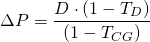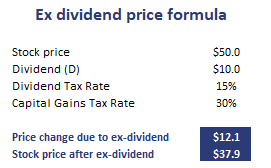# Ex dividend price formula

The ex-dividend price change measures the drop in the stock price as the stock goes ex-dividend. In fact, the share price always drops after the ex-dividend date. That’s because money is leaving the company and thus the investor’s ownership in the company is worth less. Fortunately, there is an ex dividend price formula that allows us to calculate the change in the share price.

On this page, we discuss how to calculate the ex-dividend price. We also apply the formula using an example. The ex dividend share price calculator is available for download at the bottom of the page.

## Ex dividend price formula

Let’s start by presenting the formula:where P is the price of the stock, D is the dividend, TD is the tax on dividends and TCG is the tax on capital gains. Hence, we can perfectly anticipate the drop in the stock’s share price if we know the size of the dividend, and the tax rate on dividends and capital gains.

Let’s apply the formula to some actual data to get a better understanding.

## Ex dividend share price calculation

Let’s discuss how to calculate the ex dividend share price using an actual example. The following table shows a simple example. let’s assume the company pays out \$10 in dividends. The capital gains tax rate is 15% and the dividend tax rate is 30%.The spreadsheet used to create an ex dividend price calculator is available at the bottom of the page.

## Summary

We discussed the calculation of the drop in the share price after the stock goes ex-dividend. We can easily predict the drop in the stock price using a simple formula. This shows that the share price drop can easily be anticipated and is not something that is unpredictable.

But it’s not because we know the drop in the share price, that we can make a profit from this effect. Market participants will anticipate this price change.